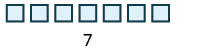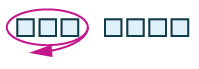Subtracting Whole Numbers: Notation and Modeling

Learning Outcomes

• Use symbols and words to represent subtraction
• Translate subtractions from math notation to words
• Use base-10 blocks to model subtraction

Use Subtraction Notation

Suppose there are seven bananas in a bowl. Elana uses three of them to make a smoothie. How many bananas are left in the bowl? To answer the question, we subtract three from seven. When we subtract, we take one number away from another to find the difference. The notation we use to subtract $3$ from $7$ is

$7 - 3$

We read $7 - 3$ as seven minus three and the result is the difference of seven and three.

Subtraction Notation

To describe subtraction, we can use symbols and words.

Operation Notation Expression Read as Result
Subtraction $-$ $7 - 3$ seven minus three the difference of $7$ and $3$

example

Translate from math notation to words:

1. $8 - 1$
2. $26 - 14$

Solution

1. We read this as eight minus one. The result is the difference of eight and one.
2. We read this as twenty-six minus fourteen. The result is the difference of twenty-six and fourteen.

try it

The video below provides more examples of how to translate subtraction word phrases.

Model Subtraction of Whole Numbers

A model can help us visualize the process of subtraction much as it did with addition. Again, we will use $\text{base - 10}$ blocks. Remember a block represents 1 and a rod represents 10. Let’s start by modeling the subtraction expression we just considered, $7 - 3$.

 We start by modeling the first number, $7$.Now take away the second number, $3$. We’ll circle $3$ blocks to show that we are taking them away.Count the number of blocks remaining.There are $4$ ones blocks left. We have shown that $7 - 3=4$ .

example

Model the subtraction: $8 - 2$

try it

Model: $9 - 6$

Model: $6 - 1$

example

Model the subtraction: $13 - 8$.

As we did with addition, we can describe the models as ones blocks and tens rods, or we can simply say ones and tens.

try it

Model the subtraction: $12 - 7$

Model the subtraction: $14 - 8$

example

Model the subtraction: $43 - 26$

try it

Model the subtraction: $42 - 27$

Model the subtraction: $45 - 29$

Watch the video below to see more examples of using base 10 blocks to model subtraction.